4. Analytical techniques

4.1 IR Spectroscopy

Infrared spectroscopy works by having a beam of infrared light absorbed by a sample. This excites different molecules and different vibrational modes at selected wavenumbers. Each of these wavenumbers has an associated energy given by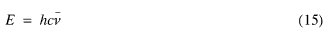where h is Planks constant and c is the speed of light (taken as 2.98 x 108 m s-1). This is energy (Joules) per molecule.

Energy is normally measured in kiloJoules per mole (kJ mol-1). (15) must be changed to accommodate this: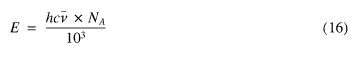Wavenumbers are also in cm-1. The SI unit of length is the meter. (16) must again be changed by multiplication by 100, if c is in m s-1.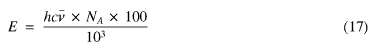The excitation wavelengths are measured by the transmitted residual light detected by the spectrophotometer and give rise to relatively sharp bands. Each of these bands has a significance.

For example, a band at approximately 2900 cm-1 is due to the absorption of an -NH2 group, whereas one at 382 cm-1 is due to an -NI group1.

As with any light absorption, the concentration of the material in question can be calculated by use of the Beer Lambert law (17), given the cell path length.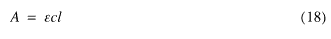where A is the absorbance, e is the extinction coefficient and l is the path length of the cell.

The e values are available from prior calibration using a graph of absorbance vs. a series of prepared (known) concentration solution of the NI3 adduct. e is then the gradient of the "best fit" line drawn through the data points produced.

4.1.1 IR cells (flats)

There are two types of i.r. window materials suitable for broad range analysis, sodium chloride and potassium bromide.

Sodium chloride cells are suitable for most compounds which have bands between 4000 and 800 cm-1. Below this point, the sodium chloride absorbs the light, thus making it useless for any analysis under 800 cm-1. The main reason for the popularity of this type of cell is because they are cheap, easy to make and simple to use.

For analysis of wavenumbers below 800 cm-1, potassium bromide flats are used. These give reproducable results to 225 cm-1 before the bromide starts to effect the readings in the same way sodium chloride flats do below 800 cm-1.

Nitrogen triiodide typically absorbs between 600 to 295 cm-1 with varied absorbances due to rotation and polymeric structures. The polymeric structures (the mono and tri-ammonical species) will have slightly different energies. These will cause some slight interference between each other due to rotations within the structures (the real structure is not known by i.r.).

4.2 X-Ray diffraction (XRD)

XRD is a method of determining structure by the difference in X-Ray interference patterns. These interferences give a set of camera angles which can be analysed using the Bragg equation (19), and the results treated by a suitable package (such as ORTEP - Oak Ridge Thermal Ellipsoid Program) for the final structure.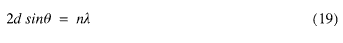The significance of each factor for equation (19) are shown in diagram 86.

6  Brown, G.I. Introduction to Physical Chemistry, 3rd Ed. p. 165. Longman 1994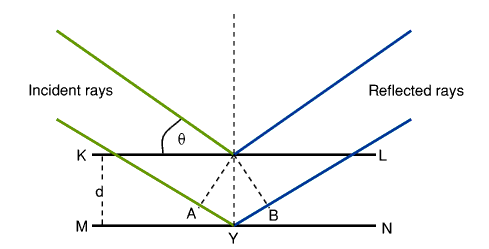Diagram 8. Significance of figures contained within equation (19)

The atoms are in a crystal are arranged in a series of planes (represented by KL and MN in diagram 8). When a beam of X Rays is incident at a glancing angle of q, some reflection takes place and diffraction is caused by interference of the reflected rays. The path difference between the reflected rays from those of KL and from MN is equal to AYB. Taking d to be the distance between the two planes, AY is therefore equal to dsin q. For the reflected rays to reinforce, the necessary condition is that AYB equals an integral number, n, wavelengths, l.

This method tends to be very time consuming (each set of results can take almost 12 man hours to analyse, interpret and compute). The results can be spoilt by any slight vibration, atmospheric pressure, changes in temperature, out of date photographic materials , processing faults and so on.

4.3 UV/VIS Spectroscopy

Ultra-violet / visible (UV/VIS) spectroscopy works in the same way as i.r. spectroscopy where a beam of light with a selected wavelength is passed through a sample. The amount of light absorbed at the differing wavelengths is plotted and an absorption vs. wavelength graph can be plotted.

As with i.r. spectroscopy, the cell used is of great importance. There are three types readily available, quartz, glass and plastic.

Plastic cells are cheap, give consistent results and can be used with a good number of solvents. They cannot be used with organic solvents or at wavelengths of below 300nm as the plastic absorbs in the u.v. region.

Quartz cells are expensive and give consistent results. They have the advantage of being able to withstand organic solvents and can be used for study in the u.v. regions. Glass also has some of the properties of quartz, but should not really be used under » 300nm.

Nitrogen triiodide is insoluble in inorganic solvents (such as water and ammonia) but is soluble in organic solvents. In aprotic solvents (such as pyridine), NI3 is measurable. The need for an organic solvent means glass or quartz cells are required.

Any aprotic solvent containing nitrogen (such as pyridine or pyrrole, but not piperidine - this is fully saturated which means it will not form the triiodide adduct) should be able to stabilise the NI3 as NI3.py with only a single pyridine molecule. There have been theories that this has been due to the relative sizes of pyridine in comparison with that of NH3 in either the mono or tri ammoniate, but it is more likely that the NI3 will dissolve in the solvent in preference to forming the adduct. Dissolution here is followed by a gradual decomposition of the NI3 and dispersion of the iodine in the solvent.